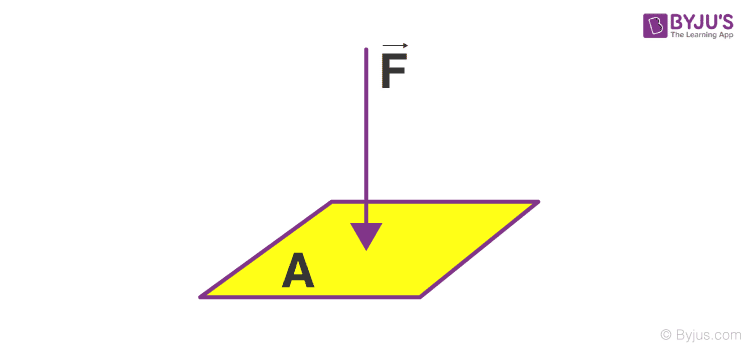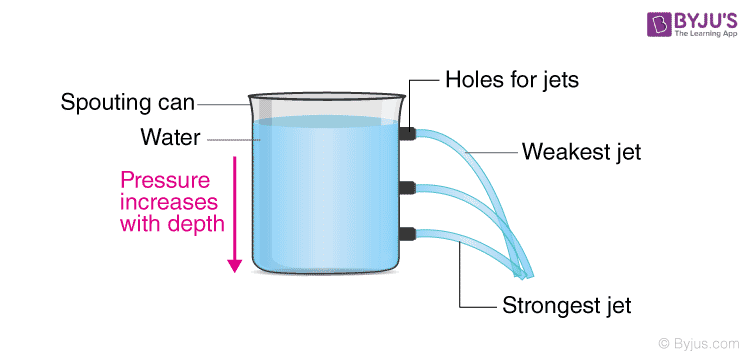# Hydrostatic Pressure And Fluid Pressure

## Introduction

Have you ever wondered why our knives need to be so sharp or why the nails we use the end with a sharp point? The answers to all these questions lie in the concept of pressure. The pressure is the ratio of the force applied to the surface area over which the force is applied. Hydrostatic pressure is the pressure exerted by liquids. When a force of ‘F’ Newton is applied perpendicularly to a surface area ‘A’, then the pressure exerted on the surface by the force is equal to the ratio of F to A.

Hence, the formula for pressure (P) is:

 $$\begin{array}{l} \frac FA \end{array}$$There are various units to describe Pressure some of which we will discuss further in this article.

• The SI Unit of Pressure is the Pascal (Pa).
• A Pascal can be defined as a force of one newton applied over a surface area of one square meter. Though pressure is exerted by solid objects, the pressure exerted by fluids is the most curious. Fluid here refers to both liquid and gases, substances that take the shape of their container.
• Fluid pressure refers to the pressure exerted by both liquids and gas

## What Is Fluid Pressure?

Firstly solid objects do not change their shape on the application of pressure which is obviously not the case with fluids. Fluid pressure can be caused by gravity, acceleration or forces when in a closed container. Since a fluid has no fixed shape, it acts equally in all directions. When you fill water in a bottle, the weight of the water inside is acting on all the sides of the bottle equally.

This force is always applied perpendicularly to the surface of the container. You can see this in a balloon. As you fill the balloon with air, you will notice that the balloon expands uniformly, all the sides blow up equal mounts and no one side is inflated more than the other. This phenomenon is also exhibited by liquids in a container

## What Is Hydrostatic Pressure?

The hydrostatic pressure is the pressure exerted by a fluid at equilibrium at any point of time due to the force of gravity. Hydrostatic pressure is proportional to the depth measured from the surface as the weight of the fluid increases when a downward force is exerted.
Fluids exert pressure in all directions equally. This rule leads to another interesting occurrence. If we consider the layer of water on the top of a bottle, the pressure exerted by the layer of water acts on the container at the sides, the surface of air on top and the layer of water at the bottom. As we move down from the top of the bottle to the bottom, the pressure exerted by the top layer on the bottom adds up.
Due to this phenomenon, the fluid at the bottom of the container experiences more pressure that the fluid which is above it.Holes at different heights in the container can be used to display the concept of Hydrostatic Pressure. The streams from the lower holes flow with a greater velocity due to the pressure from the fluid above it.

### Experiment to Understand Hydrostatic Pressure and Fluid Pressure

The pressure difference can be seen in a simple experiment. Fill up a bottle with water and take a pin to poke holes in the bottle. Now poke a hole on the top of the bottle near the surface of the water. The water dribbles out slowly from this hole. Now start moving down and making holes. As you move down, the speed and the distance travelled by the stream of water coming out of the hole increases

The speed and the distance of the outlet are maximum close to the bottom. The water pours out of the hole due to the pressure acting on the container by water in all sides. The pressure acting on the water decides the speed and the distance of the stream and at a particular height it is constant, as in if multiple holes are made in the bottle at the same height, then the flow of water from all the holes will be equal.

#### Observation:

Also, another curious observation here is that pressure acting on a layer of fluid in the bottom is proportional to the product of the height of fluid above it to its density. The hydrostatic pressure is caused in fluids mainly because of the gravitational force acting on every layer of the fluid.

This property of fluid pressure means that the pressure at the deep portions of the oceans are truly enormous, and they are. With every 10 meters, you go into the ocean, an extra 1 kilogram of pressure is added per square centimetre of your body. After a certain depth, the pressure becomes high enough to crush a human being if they are not wearing special equipment. These properties hold true for gases as well which we will explore in our article on the atmospheric pressure.